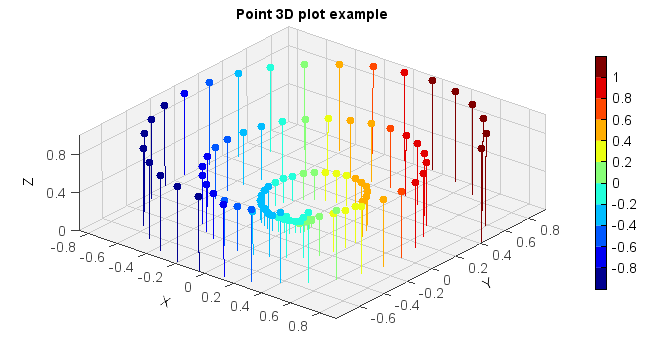# MeteoInfo 1.5 was released (2018-7-9)¶

• Update BIL data reading function.

• Update console display functions.

• Update netCDf files join function.

• Add linint2 function to interpolate from a rectilinear grid to another rectilinear grid using bilinear interpolation.

• Add rh2dewpoint function to convert relative humidity to dew point.

• Support AMap as web map.

• Support MICAPS 131 data format.

• Update geotiff read function.

• Add gridshow and stem plot function.

• Add functions to plot multi color lines.

• Read HYSPLIT trajectory data as 2D array.

3D stem plot:

z = linspace(0, 1, 100)
x = z * np.sin(20 * z)
y = z * np.cos(20 * z)
c = x + y

#Plot
ax = axes3d()
points, stemlines = ax.stem(x, y, z, c=c, edge=False, samestemcolor=True)
colorbar(stemlines,shrink=0.8)
title('Point 3D plot example')